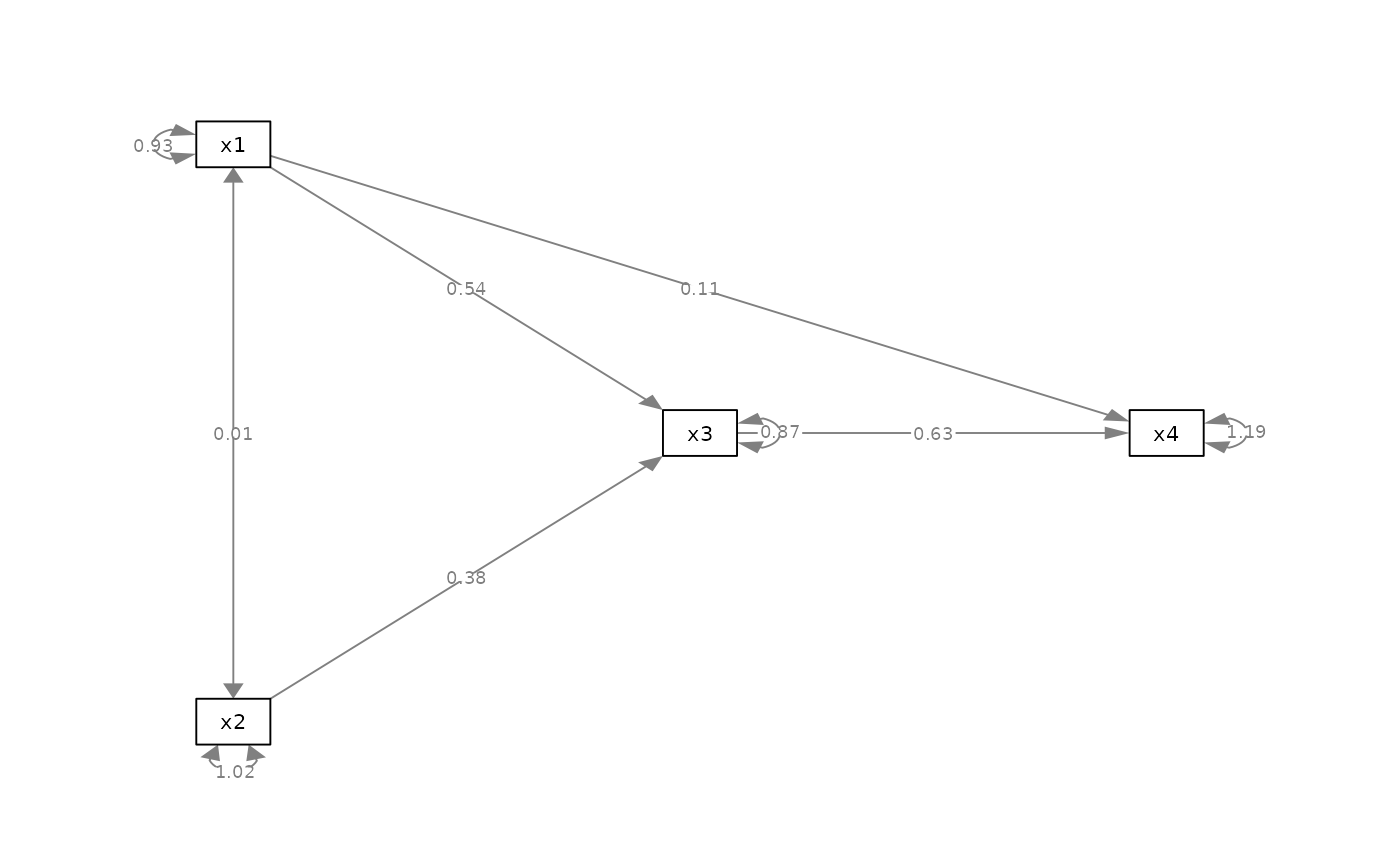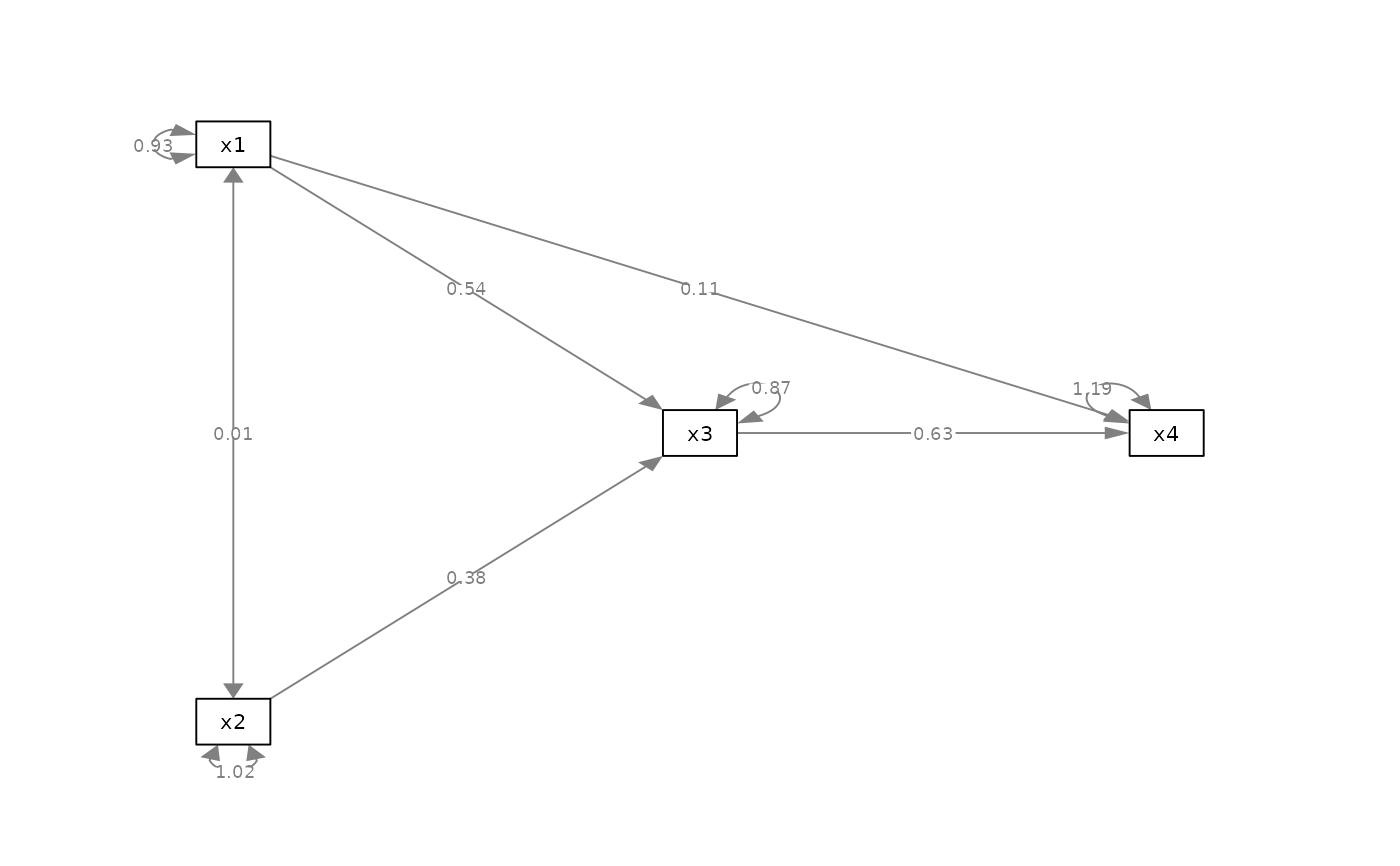Rotate the residuals of selected nodes.

## Usage

rotate_resid(semPaths_plot, rotate_resid_list = NULL)

## Arguments

semPaths_plot

A qgraph::qgraph object generated by semPlot::semPaths, or a similar qgraph object modified by other semptools functions.

rotate_resid_list

A named vector or a list of named list. For a named vector, the name of an element is the node for which its residual is to be rotated, and the value is the degree to rotate. The 12 o'clock position is zero degree. Positive degree denotes clockwise rotation, and negative degree denotes anticlockwise rotation. For example, c(x3 = 45, x4 = -45) means rotating the residual of x3 45 degrees clockwise, and rotating the residual of x4 45 degrees anticlockwise. For a list of named lists, each named list should have two named values: node and rotate. The position of the residual of node will be placed at rotate, in degree. For example, list(list(node = "x3", rotate = 45), list(node = "x4", rotate = -45)) is equivalent to c(x3 = 45, x4 = -45).

## Value

A qgraph::qgraph object based on the original one, with loopRotation attributes of selected nodes modified.

## Details

Modify a qgraph::qgraph object generated by semPlot::semPaths and rotate the residuals of selected nodes. Currently only supports "ram" and similar styles of semPlot::semPaths.

## Examples

mod_pa <-
'x1 ~~ x2
x3 ~  x1 + x2
x4 ~  x1 + x3
'
fit_pa <- lavaan::sem(mod_pa, pa_example)
lavaan::parameterEstimates(fit_pa)[, c("lhs", "op", "rhs", "est", "pvalue")]
#>   lhs op rhs   est pvalue
#> 1  x1 ~~  x2 0.005  0.957
#> 2  x3  ~  x1 0.537  0.000
#> 3  x3  ~  x2 0.376  0.000
#> 4  x4  ~  x1 0.111  0.382
#> 5  x4  ~  x3 0.629  0.000
#> 6  x3 ~~  x3 0.874  0.000
#> 7  x4 ~~  x4 1.194  0.000
#> 8  x1 ~~  x1 0.933  0.000
#> 9  x2 ~~  x2 1.017  0.000
m <- matrix(c("x1",   NA,   NA,
NA, "x3", "x4",
"x2",   NA,   NA), byrow = TRUE, 3, 3)
p_pa <- semPlot::semPaths(fit_pa, whatLabels="est",
style = "ram",
nCharNodes = 0, nCharEdges = 0,
layout = m)my_rotate_resid_vector <- c(x3 = 45, x4 = -45)

p_pa2v <- rotate_resid(p_pa, my_rotate_resid_vector)
plot(p_pa2v)my_rotate_resid_list <- list(list(node = "x3", rotate =  45),
list(node = "x4", rotate = -45))

p_pa2l <- rotate_resid(p_pa, my_rotate_resid_list)
plot(p_pa2l)